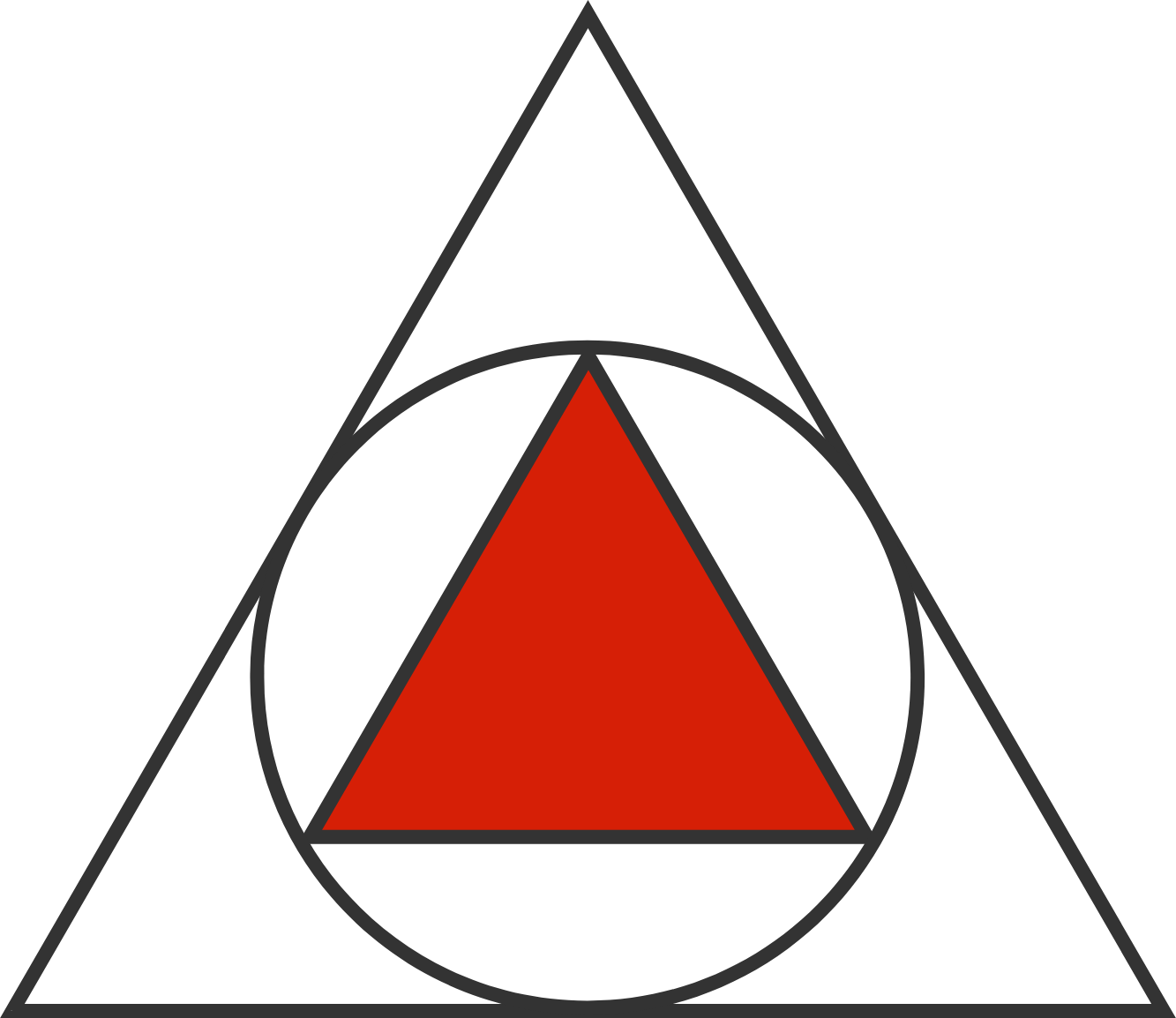# Triangle in Circle in Triangle

Geometry Level 1In a large equilateral triangle, we draw the incircle. We then draw a smaller equilateral triangle in the incircle.

What is the ratio of the area of the smaller equilateral triangle to that of the larger equilateral triangle?

×

Problem Loading...

Note Loading...

Set Loading...